# The Harmonious Mathematics of Music

According to legend, the Ancient Greek Pythagoras was once walking on the streets of Samos, when the sounds of blacksmiths’ hammering suddenly gave him an epiphany. Pythagoras rushed into the shop and, as he analyzed mathematically the shapes of the blacksmiths’ hammers, he laid the foundations of music that today’s Rihanna, Shakira and others are building upon.

Wait a minute… Are you talking about the guy from the Pythagorean theorem?

I am. And despite what you’ve learned at school, this Pythagorean theorem is absolutely not Pythagoras’ greatest idea — most notably because he probably wasn’t even the one who came up with the Pythagorean theorem. At least, that’s what I think: Pythagoras’ greatest idea was the mathematization of music, whose harmonious structures would later be the playground of the greatest musicians like Ludwig van Beethoven (we’ll get there!), or like the brilliant mathemusician Vi Hart:

I’ve been a bit reluctant about writing this article at first because I’m very, very bad in music. I have never taken any music class, I play no instrument and I sing horribly false — seriously, do not ask me to sing! But I do enjoy listening to music… especially since I’ve found out about the fundamental mathematical structures of mathematics! I guess that it’s an amazing example of how mathematics has allowed me to greatly appreciate an art I never paid enough attention to!

## The Perfection of Octaves

As the story goes, when Pythagoras started to play with hammers, he noticed that two of them were particularly harmonious with respect to one another. He measured the weights of these hammers and found out something absolutely startling.What did he find out?

The heavier of the two hammers was exactly twice the weight of the lighter one! Exactly twice.

Exactly twice? How come?

I know! What were the odds? Pythagoras had focused on these hammers solely because of musical aesthetic considerations. And yet, out of this personal taste of musical harmony, emerged a perfect ratio of 2.

Okay, actually, this beautiful story is probably apocryphal… But is there a Pythagoras’ story that’s not apocryphal?
That is very surprising… and intriguing!

I know! In fact, it was so neat that Pythagoras went on claiming that whole numbers ruled the world… which apparently led his “philosophy school” to drown Hippasus of Metapontum, because Hippasus was claiming to have found some non-perfect-ratio number in the supposedly perfect realm of geometry!

Find out more with my article on numbers and constructibility.
So, is there an explanation of this ratio of 2?

To understand this ratio, Pythagoras turned his attention to the simplest musical instrument: A string. Could it be that the ratio of 2 applied to the string also allow for some musical harmony? Is there harmony between the vibration of the string and, say, the vibration of half of the string?

Is there?

There is. Today, this essential harmony is called an octave. In fact, octaves are so important in music that we gave the same name to two notes separated by an octave. You know? $A$, $B$, $C$, $D$, $E$, $F$, $G$… Two $C’s$ are separated by exactly an octave. If they are notes played by a string, one corresponds to a string exactly twice the length of the other.Okay, it was a small lie. Actually, what matters isn’t the length of the string, but the frequency at which it vibrates. The greater this frequency, the higher-pitch the note. It turns out that for most (musical) strings, it’s a good approximation to say that a string half the length will vibrate twice as fast.
But is there a reason why two notes separated by an octave sound harmonious?

There is! Let’s take the longer string. Let’s say it plays a $C$. It will be a low-pitch $C$. Now, if you pinch the string in the middle, the string will vibrate in a sort of perfectly symmetric way.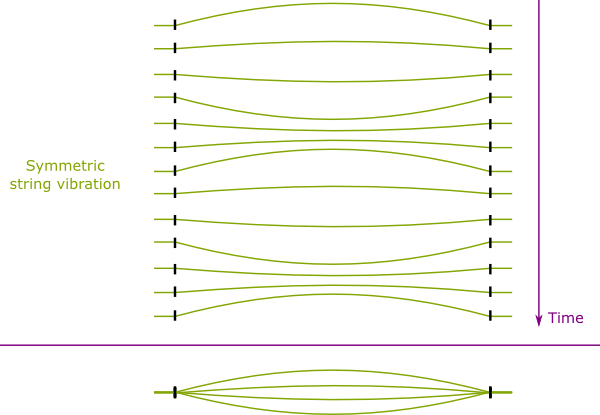So far so good.

But if you don’t pinch it in the middle, it will vibrate in a much more complicated way. A very nastily complicated way. But there’s one thing we do know about the vibration of the string…

What is it?

End points of the string are fixed. And that’s very important.

Why?

The only symmetric vibrations that leave end points fixed are vibrations that perfectly divide the string in portions of equal lengths. These are called harmonics of different modes. And amazingly, we can prove mathematically that any asymmetric vibration of the long string is the sum of the vibrations of the harmonics.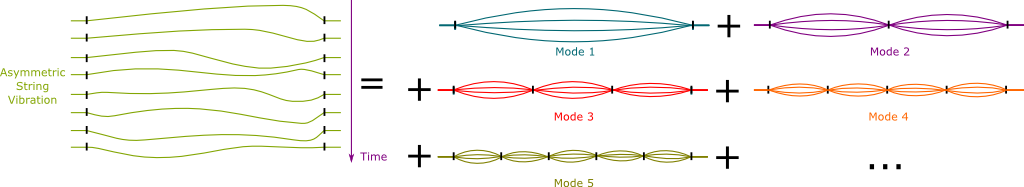This decomposition is one of the most remarkable fact of mathematics! It is called the Fourier decomposition, and it turned out to be an essential component of many theories, from the ones that explain how our ears can hear and enjoy music, to how matter is distributed throughout our universe, and to the fundamental physics of elementary particles!

Now, as you can imagine, unless you pinch the string at its extremity, the higher harmonics will not be loud enough to be heard. In fact, we’ll mainly hear the harmonics of modes 1 and 2.

I get it! Mode 2 is precisely the higher octave?

Exactly! And that’s the reason why two $C’s$ sound so harmonious: The low-pitch $C$ also “plays” the higher-pitch one. It sort of contains it, and thus, when we move from one to another, our ears detect some harmonious continuum.

In fact, in our ears, the different notes are heard by tiny hair. Each hair is sensitive to a specific pitch. By moving from a do to another, some of the hair will carry on their motions, and this is what explains the harmony that our brain then feels.

## Let’s Create the Notes

Evidently, if music was only made of octaves, it’d be a bit boring. It’d be pleasing, but a bit monotone. To go further we need to create new notes. And we’ll do that solely based on requirements of harmony.

What do you mean by “creating new notes”?

Let’s consider one string of reference, and let’s say it plays the fundamental note called $0$. We’ve seen that by halving the length of the string, we could define other notes in perfect harmony with the fundamental $0$. And as we’ve said it, these other notes that differ from $0$ by octaves are called $0’s$ as well. More formally, taking higher and lower octaves define all the notes $0’s$ of music.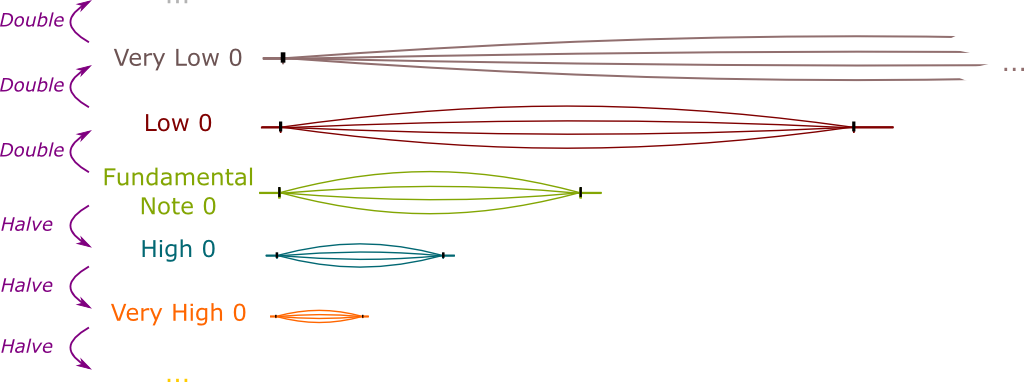But music isn’t made only of $0’s$. We need more notes. How can we create them through a coherent mathematical process?

What about dividing the string in thirds?

Bingo! As we’ve seen earlier, when $0$ is played, we hear all its harmonics. Moreover, the harmonics that we will hear the most will be those of smaller modes. Now, as we’ve seen modes 1 and 2 are $0’s$. Next is mode 3. This mode 3 harmonic is not any octave higher, so it is not a $0$. It has to be a new kind of note. Let’s call it the note $1$.Crucially, the note $1$ is contained within $0$. When $0$ is played, we hear $1$. And that’s why the two notes are harmonious. Musicians say that $1$ is the perfect fifth of $0$.

You’ve been saying that notes separated by octaves or perfect fifths sound harmonious… But why should I believe you?

Let’s listen to this video. The author of the video first plays a series of octaves, and then a series of perfect fifths.

Okay, that sounded good indeed. But why are perfect fifths called perfect fifths?

I’ll get to that later (I personally would rather call it a perfect third…). Now that we have one new note $1$, we can also create all notes $1’s$! How?

They’re octaves higher and lower of the $1$ we’ve created!

Exactly!

Cool! But then, can’t we create perfect fifths of $1’s$?

It’s an awesome observation you’ve just made. In fact, that’s how we can create all the notes of music: We just make up the perfect fifth of the last note we’ve created, and its octaves as well! Thereby, we construct $2$ as the perfect fifth of $1$, $3$ as the perfect fifth of $2$, and so on.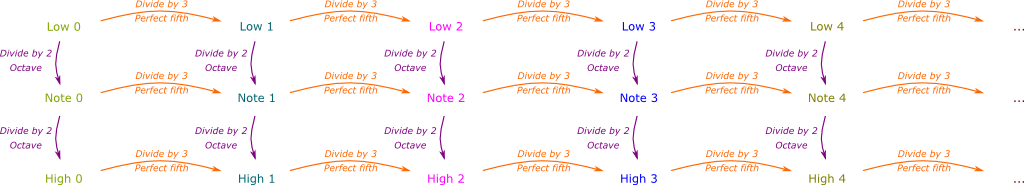As you’ve noticed, I’ve stopped drawing the strings the notes correspond to. That’s because the strings of high-pitch notes are much much smaller than those of low-pitch ones. And the reason for that is that all these multiplicative processes necessarily range on huge scales. Such scales cannot be represented all at once on a figure. That’s why, instead, we use so-called logarithmic scales that allow to contract scales by replacing multiplications by additions. That’s why all $C’s$ are equally spaced on a piano keyboard. What separates them is described in terms of additive variations, rather than multiplicative ones.
Doesn’t this creation process stop?

In principle, it shouldn’t stop. We should be creating infinitely many notes!

Are you crazy? How can we compose music if there are infinitely many notes?

That’s a good point.

## The Impossibility Theorem of Music

We should have a finite number of notes. But that can only happen if some note $n$ we’ve created is the “same” as the note $0$. More precisely, $n$ must be is some octaves higher than $0$.

Indeed, if $n$ is the same as $0$, then any $n+k$ is the same as $k$. Hence, all the notes are actually between $0$ and $n-1$, and thus there are only $n$ notes. I’ll let you prove the reciprocal as an exercise, namely, if there are a finite number notes, then there must be some note $n$ that is the same as note $0$.

Unfortunately, it’s not too hard to prove mathematically that this can’t be. This is what could be called the impossibility theorem of music: Perfect harmony in music requires an infinite number of musical notes.

Why is that?

Any note we’ve created is obtained by repeatedly taking thirds. In fact, the note $n$ corresponds to a string of length $1/3^n$ of the original string. Moreover, its $k^{th}$ lower octave is obtained doubling $k$ times the length of the string. Thus, the $k^{th}$ lower octave of note $n$ corresponds to a string length that is $2^k/3^n$ of the original string.

I see! So, for this note to be the same as the original note, we must have $2^k/3^n = 1$, right?

Exactly! In other words, we must have $2^k = 3^n$. But unfortunately, this can’t be. This equation has no solution.

How do you know?

The left term $2^k$ is even but $3^n$ is odd. An even number can’t be odd…

Good point… So, what? Are we screwed?

Before telling you what we can do, let me state the impossibility theorem of music.More precisely, any note is physically defined by a frequency of vibration. Suppose we have one frequency of reference. Then other notes can be defined by how much greater (in ratio) they are. In other words, we can regard the set of notes as the set $\mathbb R_+^*$ of strictly positive real numbers. We then have the “separated-by-octaves” equivalence relation between string length, defined by $x \sim y$ if there exists $k \in \mathbb Z$ such that $x = 2^k y$. Let’s call $\mathsf{Notes}$ the quotient space $\mathbb R_+^* / \sim$. Then, we have a function $\mathsf{PerfectFifth} : \mathsf{Notes} \rightarrow \mathsf{Notes}$, defined by $\mathsf{PerfectFifth}(x) = x/3$. Then, any subset $\mathsf{MusicalNotes} \subset \mathsf{Notes}$ stable by $\mathsf{PerfectFifth}$ (i.e. such that $\mathsf{PerfectFifth} (\mathsf{MusicalNotes}) \subset \mathsf{MusicalNotes}$) is infinite.

## The Fundamental Theorem of Music

Here’s a positive breaking news though. Despite the impossibility theorem, musicians still manage to play pretty good music.

So how did they find harmony in musical notes?

Do you know how many notes there are in an octave?

Well, there’s $A$, $B$, $C$, $D$, $E$, $F$ and $G$. So, seven!

Are you sure? Aren’t you forgetting some?

Oh yeah! There’s also $A\#$, $C\#$, $D\#$, $F\#$ and $G\#$. That’s a total of twelve notes!

It is, isn’t it? This means that something has happened when the note $12$ has been created. This note $12$ corresponds to dividing the string length by $3^{12}$. This is $3^{12} = 531441$. Yet, we also have $2^{19} = 524288$. The ratio of these equal $2^{19}/3^{12} \approx 0.987$. That’s almost 1!

I see! So note $12$ is almost a $0$!

Exactly. In fact, I’d call the approximate equality $2^{19} \approx 3^{12}$ the fundamental theorem of music. Stated differently, it says that the note $12$ is nearly the same as $0$. And all of music has built upon this fundamental approximation, sometimes known as the Pythagorean comma.We could have done better! Indeed, we could have used the approximation $2^{84}/3^{53} \approx 0.998$ to define 53 notes. It’s even possible to do better, and the reason for that lies in the irrationality of $\ln(3)/\ln(2)$. More precisely, using the logarithm the set $\mathsf{Notes}$ with multiplication can be shown to be isomorphic to the Lie group of the circle $\mathbb R / \mathbb Z$, and the perfect-fifth operator then corresponds to the translation by $\ln(3)/\ln(2)$ in the circle group. Ergodic theory then proves that the irrationality of $\ln(3)/\ln(2)$ shows that the translation is mixing. In particular, by repeating the perfect-fifth operator, we can get arbitrary close to the original point (this can also be proved using Poincaré’s recurrence theorem). But somehow, musicians seem to consider that 53 notes would already be slightly too many and that $0.987$ is already close enough to $1$… They’re even worse than physicists!

Now that we have 12 notes, we should all order them not only according to harmony as we have done, but according to how they fit in a single octave. I spare you the computations, but we can see that the note of slightly higher pitch than $0$ is $7$, then $2$, $9$, $4$, $11$, $6$, $1$, $8$, $3$, $10$, $5$. And then we’re back at $0$.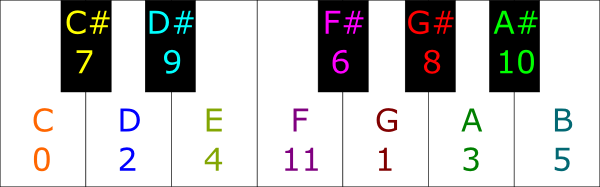Because of the Pythagorean comma, the note following $5$ should be $12$, which is slightly under the higher $0$. To avoid the abrupt slightly distinguishable gap between $12$ and $0$, piano tuners usually spread the Pythagorean comma within every perfect fifth. More precisely, the difference between successive notes, which theoretically should be $2^8/3^5$, is defined as $\sqrt{2}$. Fortunately, this slight difference between $2^8/3^5$ and $\sqrt{2}$ is not audible to the untrained ear! Finally, the fact that the difference between any two successive notes is the same means that there is a sort of homogeneity in the notes. We can shift all songs by a ratio $\sqrt{2}$ and all (multiplicative) distances between notes, which is what the untrained ears only hear, would be the same.
That sequence $0$, $7$, $2$, $9$, $4$, $11$, $6$, $1$, $8$, $3$, $10$, $5$… It looks like a random list of numbers!

Look carefully. It’s not a random list at all. There’s a neat underlying structure. From a note to the next one, either you add 7 or you subtract 5.

More precisely, you always add 7, but you subtract 12 when the number you obtain exceeds 12: The sequence is an arithmetic progression with common difference 7, in the set of numbers modulo 12. In other words, it’s the sequence $7k \; mod \; 12$ Since 7 and 12 are coprime, this sequence is surjective of rank 12.

Now, this gives us two different ways of ordering the notes of music. We can either order them by pitch proximity in an octave, or we can order them by harmony of perfect fifth: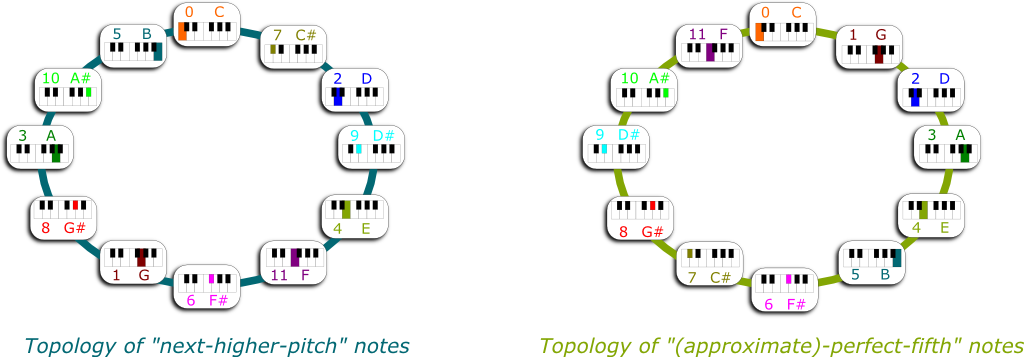In fact, musical notes cannot be ordered. Indeed, you cannot say that $C\#$ is “higher” than $C$, because the low $C\#$ is certainly not higher than the high $C$. You can say that it is the “next” note. But as we move on to “next” notes, like $D$, $D\#$, and so on, we eventually come back to $C\#$. So it’s not much of an order relation. Mathematically, we thus do not talk about order, but about topology instead. And because by “moving on” we eventually come back to the original point, the natural topology of musical notes is the topology of a circle. But note that there are actually two topologies. One is in terms of “next higher pitch”, and the other is in terms of “perfect fifth”.

Finally, I can explain why the perfect fifth is called that way. As you can see in the figure, between the note $n+1$ is always 5 notes after the note $n$. That’s why we say that $n+1$ is the perfect fifth of $n$.

## The Second Theorem of Music

One thing that troubled me early on when I heard about music theories was the idea of chords. Some triplets of notes sound good, while others sound terrible. I always thought that this was because of our education: Good music always aline good-sounding triplets, and I thought that repeatedly hearing them led us to like them. But I’ve since stumbled upon a more fundamental explanation which I find fascinating.

Wait… What’s a nice chord?

The most famous of all chords is the major triad. An example of a major triad is the $C$ major chord. The $C$ major chord plays $C$, $E$ and $G$. This major chord is music’s most celebrated chord. And with reason. It does sound extremely harmonious, as opposed to other random choices of three notes.

So, why is it harmonious?

Hehe… Remember how a vibrating string can be decomposed into modes?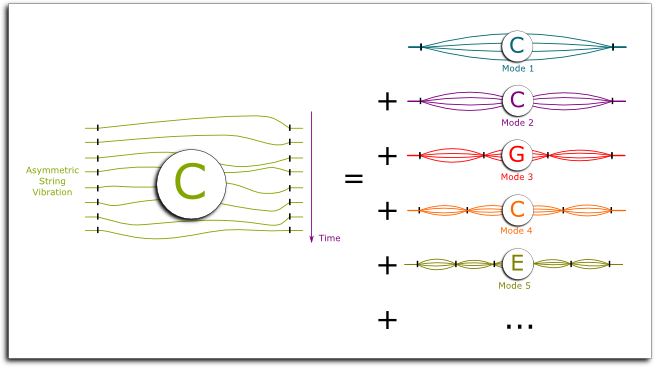Yes I remember.

So, say mode 1 is $C$. Then, mode 2 is…

the C an octave higher!

Yes! Mode 3 is…

the perfect fifth of $C$, which is $G$, right?

Very good. What about mode 4?

Another $C$ two octaves higher!

Excellent! And now… what about mode 5?

I don’t know… Wait, I see where you’re getting at… It’s $E$, isn’t it?

Humm… Is it?

Isn’t it?

Well, let’s compute it! Recall that $E$ is what we constructed as the note $4$. So, the length of the string it corresponds to is $1/3^4$ of $C$. Let’s now consider the octave 4 times higher. It corresponds to a length of $2^4/3^4$ of that of $C$. Well, $2^4/3^4 \approx 0.198 \approx 0.2 = 1/5$. Do you know what that means?

I do! It means that E is the mode 5 of the string!

Exactly!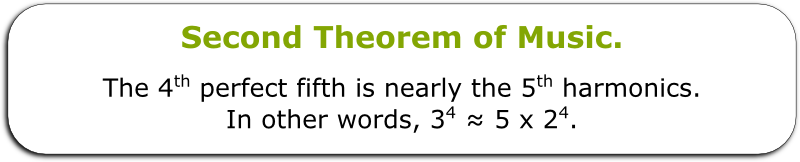In other words, when we play $C$, we also hear its modes 2, 3, 4 and 5, which corresponds to $C$, $G$, $C$ and $E$. By playing C, G and E, we are amplifying the notes that we were already hearing! That’s why the major chord is so harmonious!

Wow! This is brilliant!

I know! In fact, the intricate relationship between $C$ and $E$ has been given a name. $E$ is called the major third of $C$. This means that there are more intricate harmony relations between the musical notes, through this major-third relation.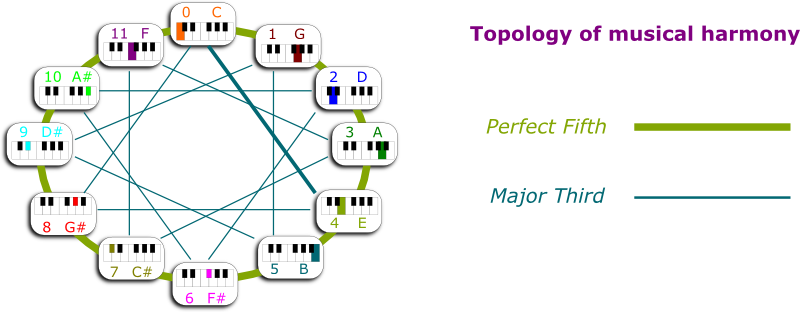It’s a tricky one. The minor chord consists of replacing the major third by another note called the minor third. The minor third of the note $0$ is the note $3$. But this minor third does not correspond to any mode. It is a bit less harmonious. But disharmony, or dissonance, may lie at the core of the compositions of the greatest musicians. At least, this is what argued for Beethoven by Natalya St. Clair in the following awesome TedEd video:

## Let’s Conclude

There’s a cliché out there according to which the mathematical study of artistic phenomena leads to some deterioration of artistic beauty. I strongly feel that this is completely false. In fact, my personal experience has repeatedly been exactly the opposite. It was rather when I learned about the underlying science or mathematics of arts that I started to appreciate artistic masterpieces the way they deserve to be appreciated. This has been totally the case with my appreciation of music. Maths only adds to the musical experience. And, for me, it adds a lot!

Really?

I’m telling you! But if you don’t trust me, please listen to the scientist that probably conveyed this idea the best, the great Richard Feynman:

Similarly, I have times and times again been terribly excited by artistic masterpieces whose mathematical structures I could understand. This was especially the case when I stumbled upon a piece of work by François Morellet, when I discovered the fractal nature of Jackson Pollock’s paintings or Escher’s brilliant use of hyperbolic geometry. Seriously, learn the maths, and you will see the whole world through much more exciting perspectives!

## One comment to “The Harmonious Mathematics of Music”

1.Inteano says:

Well. I’m totally clueless when it comes to music, and if anything, you just proved that to me …mathematically.

I was always apprehensive of the structure of music, since I couldn’t for the life of me understand whether the notes or scales were arbitrarily selected or whether they corresponded to some fixed underlying truths or givens . Though I believe I have a “feeling” for music and can appreciate many types of music just by listening to it, almost always recognizing a “good” piece from the first few notes, I always had the impression that “perfectly” harmonious music was extremely rare in any genre and so I was wondering why that could be. I felt that in every piece of music there was something amidst the harmonies that was dis-harmonic so much so that it created within me a sort of aesthetic disappointment.

It was in search for a possible answer to this that I stumbled upon your website. You have done a very good job of simplifying as much as is probably possible, yet it still all sounds like gibberish to me. Maybe after reading your article I’m now at the point where a (musically) primitive person has just realized that the earth isn’t flat and so there’s no such thing as a perfectly flat stretch of land.

I intuitively suspect that the only thing that could lure me out of my musical cave would be to listen to a piece of music that is considered by musicians to be as close as possible to a “perfectly harmonious” piece of music with no dissonant musical acrobatics so that I could start the structural comparisons from there.

Thanks for the effort , anyway ! It’s not your fault some of us are musically or mathematically retarded ….